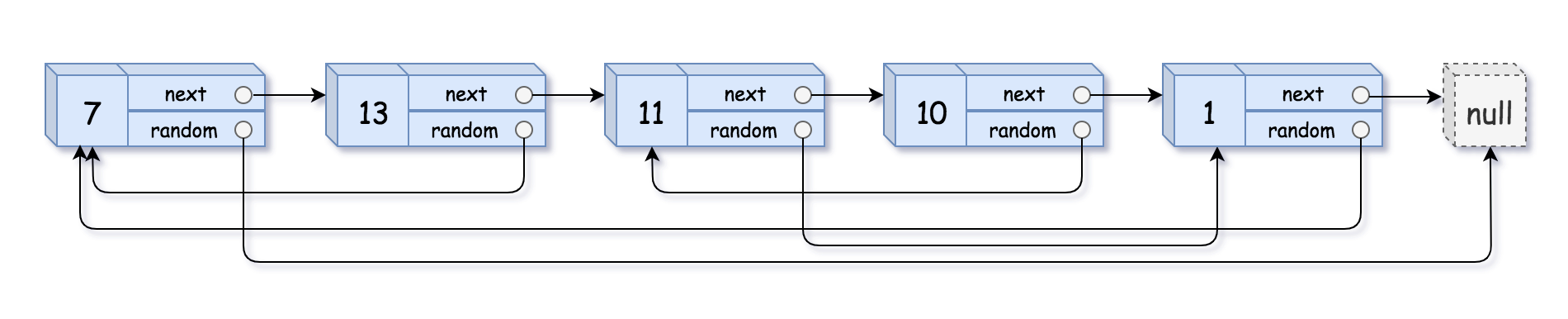# 138. Copy List with Random Pointer 复制带随机指针的链表

@TOC

## # 题目描述

• `val`：一个表示 `Node.val` 的整数。
• `random_index`：随机指针指向的节点索引（范围从 `0``n-1`）；如果不指向任何节点，则为 `null```````输入：head = [[7,null],[13,0],[11,4],[10,2],[1,0]]

````````````输入：head = [[1,1],[2,1]]

````````````输入：head = [[3,null],[3,0],[3,null]]

``````

``````输入：head = []

``````

• `0 <= n <= 1000`
• `-10000 <= Node.val <= 10000`
• Node.random 为空（null）或指向链表中的节点。

## # 解题方法

Python 代码如下：

``````"""
# Definition for a Node.
class Node(object):
def __init__(self, val, next, random):
self.val = val
self.next = next
self.random = random
"""
class Solution(object):
"""
:rtype: Node
"""
nodeDict = dict()
dummy = Node(0, None, None)
while pointer:
node = Node(pointer.val, pointer.next, None)
nodeDict[pointer] = node
while pointer:
if pointer.random:
nodeDict[pointer].random = nodeDict[pointer.random]
pointer = pointer.next
return dummy.next
``````

C++ 代码如下：

``````/*
// Definition for a Node.
class Node {
public:
int val;
Node* next;
Node* random;

Node(int _val) {
val = _val;
next = NULL;
random = NULL;
}
};
*/

class Solution {
public:
Node* copyRandomList(Node* head) {
if (!head) return nullptr;
unordered_map<Node*, Node*> copyMap;
Node* moveOld = head;
Node* moveNew = newHead;
while (moveOld) {
copyMap[moveOld] = moveNew;
moveNew->next = moveOld->next ? new Node(moveOld->next->val) : nullptr;
moveNew = moveNew->next;
moveOld = moveOld->next;
}